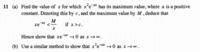# Deduce

#### burgerandcheese

##### Junior MemberHi. I need help with part (a) only because then I will be able to proceed to part (b)
I have no idea how to "deduce that xe-ax < M/x if x > c"

This is what I've done thus far:

f(x) = x2e-ax
f '(x) = xe-ax(2 - xa)
f ''(x) = e-ax(2 - 4ax + a2x2)

They are correct because I checked my answer using an online derivative calculator.

So setting f '(x) = 0 gives me x = 0 or x = 2/a
f ''(0) = 2 > 0 so f(x) is at its minimum when x = 0
f ''(2/a) = -2/e2 < 0 so f(x) is at its maximum when x = 2/a

So now c = 2/a > 0
M = f(2/a) = 4/(a2e2) > 0

How do I continue?

#### HallsofIvy

##### Elite Member
Yes, $$\displaystyle f(x)= x^2e^{-ax}$$ takes on its maximum value at c= 2/a and that maximum value is $$\displaystyle \frac{4}{a^2e^2}$$. So for x> c, in fact for any value of x other than c, $$\displaystyle x^2e^{-ax}< \frac{4}{a^2e^2}= M$$. Divide both sides of that inequality by x (which is positive).

•burgerandcheese

#### burgerandcheese

##### Junior Member
Yes, $$\displaystyle f(x)= x^2e^{-ax}$$ takes on its maximum value at c= 2/a and that maximum value is $$\displaystyle \frac{4}{a^2e^2}$$. So for x> c, in fact for any value of x other than c, $$\displaystyle x^2e^{-ax}< \frac{4}{a^2e^2}= M$$. Divide both sides of that inequality by x (which is positive).
Oh right, since M is the maximum value the inequality holds for any value other than c. Thanks